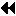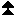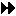Predefined reduce operations

## Predefined reduce operationsUp: Global Reduction Operations Next: MINLOC and MAXLOC Previous: Reduce

The following predefined operations are supplied for MPI_REDUCE and related functions MPI_ALLREDUCE, MPI_REDUCE_SCATTER, and MPI_SCAN. These operations are invoked by placing the following in op.

[ Name] Meaning
[ ]
[ MPI_MAX] maximum
[ MPI_MIN] minimum
[ MPI_SUM] sum
[ MPI_PROD] product
[ MPI_LAND] logical and
[ MPI_BAND] bit-wise and
[ MPI_LOR] logical or
[ MPI_BOR] bit-wise or
[ MPI_LXOR] logical xor
[ MPI_BXOR] bit-wise xor
[ MPI_MAXLOC] max value and location
[ MPI_MINLOC] min value and location

The two operations MPI_MINLOC and MPI_MAXLOC are discussed separately in Sec. MINLOC and MAXLOC . For the other predefined operations, we enumerate below the allowed combinations of op and datatype arguments. First, define groups of MPI basic datatypes in the following way.

[ C integer:] MPI_INT, MPI_LONG, MPI_SHORT,
MPI_UNSIGNED_SHORT, MPI_UNSIGNED,
MPI_UNSIGNED_LONG
[ Fortran integer:] MPI_INTEGER
[ Floating point:] MPI_FLOAT, MPI_DOUBLE, MPI_REAL,
MPI_DOUBLE_PRECISION, MPI_LONG_DOUBLE
[ Logical:] MPI_LOGICAL
[ Complex:] MPI_COMPLEX
[ Byte:] MPI_BYTE

Now, the valid datatypes for each option is specified below.

[ Op] Allowed Types
[ ]
[ MPI_MAX, MPI_MIN] C integer, Fortran integer, Floating point
[ MPI_SUM, MPI_PROD] C integer, Fortran integer, Floating point, Complex
[ MPI_LAND, MPI_LOR, MPI_LXOR] C integer, Logical
[ MPI_BAND, MPI_BOR, MPI_BXOR] C integer, Fortran integer, Byte

```

A routine that computes
the dot product of two vectors that are distributed across a
group of processes and returns the answer at node zero.

SUBROUTINE PAR_BLAS1(m, a, b, c, comm)
REAL a(m), b(m)       ! local slice of array
REAL c                ! result (at node zero)
REAL sum
INTEGER m, comm, i, ierr

! local sum
sum = 0.0
DO i = 1, m
sum = sum + a(i)*b(i)
END DO

! global sum
CALL MPI_REDUCE(sum, c, 1, MPI_REAL, MPI_SUM, 0, comm, ierr)
RETURN

```

```

A routine that computes
the product of a vector and an array that are distributed across a
group of processes and returns the answer at node zero.

SUBROUTINE PAR_BLAS2(m, n, a, b, c, comm)
REAL a(m), b(m,n)    ! local slice of array
REAL c(n)            ! result
REAL sum(n)
INTEGER n, comm, i, j, ierr

! local sum
DO j= 1, n
sum(j) = 0.0
DO i = 1, m
sum(j) = sum(j) + a(i)*b(i,j)
END DO
END DO

! global sum
CALL MPI_REDUCE(sum, c, n, MPI_REAL, MPI_SUM, 0, comm, ierr)

! return result at node zero (and garbage at the other nodes)
RETURN

```Up: Global Reduction Operations Next: MINLOC and MAXLOC Previous: Reduce